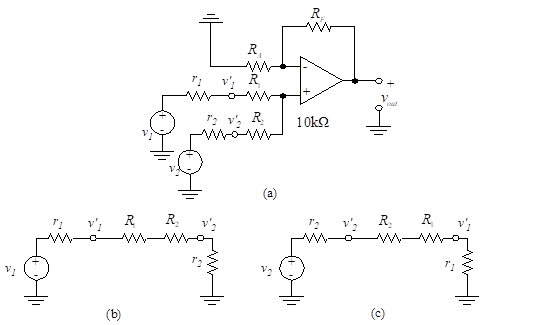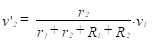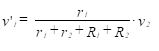# 11. Coupling between Multiple Inputs

###### Coupling between Multiple Inputs
When more than one input signal is connected to either the inverting or the non-inverting input to the op-amp, coupling between the inputs can result. This is frequently a disturbing problem, since a variation in one channel can produce an unwanted input into an adjoining channel. Consider the dual-input, non-inverting op-amp of Figure 9.47(a), where each channel is driven with a voltage source in series with a source resistance (the internal resistance of the voltage source).Figure 47 – Coupling between multiple inputs

The ideal source voltages, v1 and v2, have series resistances, r1 and r2 respectively. We begin by writing the equations for the effective voltages, v’1 and v’2, into the summing amplifier. We assume that zero current enters the op-amp. With v2 set equal to zero, as shown in Figure 47(b), the voltage into the op-amp, v’2, is(98)

When v1 = 0, the v’1 voltage is [see Figure 47(c)],(99)

Notice that the v’2 voltage comes from v1 and the v’1 voltage comes from v2. This coupling effect produces undesirable cross-talk between the two inputs. The effect can be eliminated by designing a system with r1 and r2 approaching zero. Hence, to eliminate coupling, each non-inverting multiple input should be driven with an op-amp that has zero (or very low) output impedance.